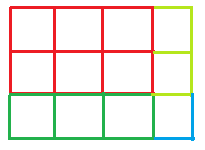GeeksforGeeks App
Open AppBrowser
Continue

# Check if it is possible to perform the given Grid Division

Given two integers N and M which represent the size of a grid. Also given an integer array arr[] of size P which represent that the given grid is divided into P parts each consisting of arr[i] cells from the grid. The task is to check whether it is possible to divide the grid in the given manner or not.
Examples:

Input: arr[] = {6, 3, 2, 1}, N = 3, M = 4
Output: YesInput: arr[] = {4, 2, 2}, N = 3, M = 2
Output: No

Approach: In order for the division to be possible, the sum of the cells of all the parts must be equal to the total number of cells in the given grid i.e. the sum of all the array elements must be equal to N * M.
Below is the implementation of the above approach:

## C++

 `// C++ implementation of the approach``#include ``using` `namespace` `std;` `// Function that returns true if it is possible to``// divide the grid satisfying the given conditions``bool` `isPossible(``int` `arr[], ``int` `p, ``int` `n, ``int` `m)``{` `    ``// To store the sum of all the``    ``// cells of the given parts``    ``int` `sum = 0;``    ``for` `(``int` `i = 0; i < p; i++)``        ``sum += arr[i];` `    ``// If the sum is equal to the total number``    ``// of cells in the given grid``    ``if` `(sum == (n * m))``        ``return` `true``;``    ``return` `false``;``}` `// Driver code``int` `main()``{``    ``int` `n = 3, m = 4;``    ``int` `arr[] = { 6, 3, 2, 1 };``    ``int` `p = ``sizeof``(arr) / ``sizeof``(arr);` `    ``if` `(isPossible(arr, p, n, m))``        ``cout << ``"Yes"``;``    ``else``        ``cout << ``"No"``;` `    ``return` `0;``}`

## Java

 `// Java implementation of the approach``import` `java.util.*;``class` `GFG``{` `// Function that returns true if it is possible to``// divide the grid satisfying the given conditions``static` `boolean` `isPossible(``int` `arr[], ``int` `p,``                          ``int` `n, ``int` `m)``{` `    ``// To store the sum of all the``    ``// cells of the given parts``    ``int` `sum = ``0``;``    ``for` `(``int` `i = ``0``; i < p; i++)``        ``sum += arr[i];` `    ``// If the sum is equal to the total number``    ``// of cells in the given grid``    ``if` `(sum == (n * m))``        ``return` `true``;``    ``return` `false``;``}` `// Driver code``public` `static` `void` `main(String[] args)``{``    ``int` `n = ``3``, m = ``4``;``    ``int` `arr[] = { ``6``, ``3``, ``2``, ``1` `};``    ``int` `p = arr.length;` `    ``if` `(isPossible(arr, p, n, m))``        ``System.out.println(``"Yes"``);``    ``else``        ``System.out.println(``"No"``);``}``}` `// This code is contributed by Princi Singh`

## Python3

 `# Python3 implementation of the approach` `# Function that returns true if``# it is possible to divide the grid``# satisfying the given conditions``def` `isPossible(arr, p, n, m):``    ` `    ``# To store the sum of all the``    ``# cells of the given parts``    ``sum` `=` `0``;``    ``for` `i ``in` `range``(p):``        ``sum` `+``=` `arr[i];` `    ``# If the sum is equal to the total number``    ``# of cells in the given grid``    ``if` `(``sum` `=``=` `(n ``*` `m)):``        ``return` `True``;``    ``return` `False``;` `# Driver code``if` `__name__ ``=``=` `'__main__'``:` `    ``n ``=` `3``;``    ``m ``=` `4``;``    ``arr ``=` `[``6``, ``3``, ``2``, ``1``];``    ``p ``=` `len``(arr);` `    ``if` `(isPossible(arr, p, n, m)):``        ``print``(``"Yes"``);``    ``else``:``        ``print``(``"No"``);` `# This code is contributed by Rajput-Ji`

## C#

 `// C# implementation of the approach``using` `System;``    ` `class` `GFG``{` `// Function that returns true if it is possible to``// divide the grid satisfying the given conditions``static` `bool` `isPossible(``int` `[]arr, ``int` `p,``                       ``int` `n, ``int` `m)``{` `    ``// To store the sum of all the``    ``// cells of the given parts``    ``int` `sum = 0;``    ``for` `(``int` `i = 0; i < p; i++)``        ``sum += arr[i];` `    ``// If the sum is equal to the total number``    ``// of cells in the given grid``    ``if` `(sum == (n * m))``        ``return` `true``;``    ``return` `false``;``}` `// Driver code``public` `static` `void` `Main(String[] args)``{``    ``int` `n = 3, m = 4;``    ``int` `[]arr = { 6, 3, 2, 1 };``    ``int` `p = arr.Length;` `    ``if` `(isPossible(arr, p, n, m))``        ``Console.WriteLine(``"Yes"``);``    ``else``        ``Console.WriteLine(``"No"``);``}``}` `// This code is contributed by Rajput-Ji`

## Javascript

 ``

Output:

`Yes`

Time Complexity: O(p)

Auxiliary Space: O(1)

My Personal Notes arrow_drop_up